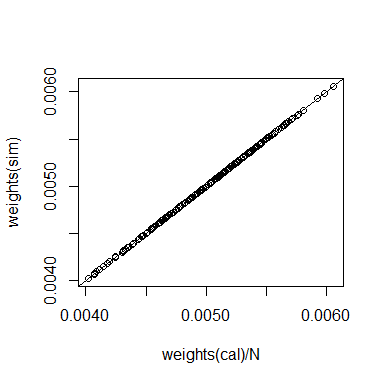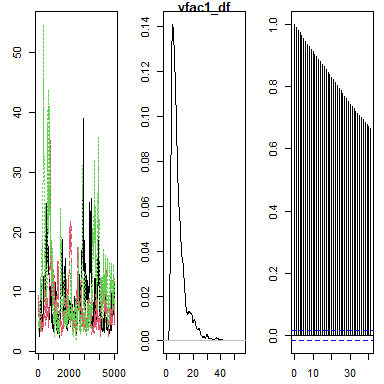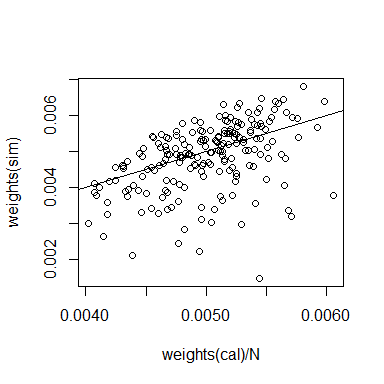# Linear regression, prediction, and weighting

We use the api dataset from package survey to illustrate estimation of a population mean from a sample using a linear regression model. First let’s estimate the population mean of the academic performance indicator 2000 from a simple random sample, apisrs. Using package survey’s GREG estimator [@Sarndal1992], we find

library(survey)
data(api)
# define the regression model
model <- api00 ~ ell + meals + stype + hsg + col.grad + grad.sch
# compute corresponding population totals
XpopT <- colSums(model.matrix(model, apipop))
N <- XpopT[["(Intercept)"]]  # population size
# create the survey design object
des <- svydesign(ids=~1, data=apisrs, weights=~pw, fpc=~fpc)
# compute the calibration or GREG estimator
cal <- calibrate(des, formula=model, population=XpopT)
svymean(~ api00, des)  # equally weighted estimate
##         mean     SE
## api00 656.58 9.2497
svymean(~ api00, cal)  # GREG estimate
##         mean     SE
## api00 663.86 4.1942

The true population mean in this case can be obtained from the apipop dataset:

mean(apipop$api00) ##  664.7126 Note that the GREG estimate is more accurate than the simple equally weighted estimate, which is also reflected by the smaller estimated standard error of the former. We can run the same linear model using package mcmcsae. In the next code chunk, function create_sampler sets up a sampler function that is used as input to function MCMCsim, which runs a simulation to obtain draws from the posterior distribution of the model parameters. By default three chains with independently generated starting values are run over 1250 iterations with the first 250 discarded as burnin. As the starting values for the MCMC simulation are randomly generated, we set a random seed for reproducibility. The results of the simulation are subsequently summarized, and the DIC model criterion is computed. The simulation summary shows several statistics for the model parameters, including the posterior mean, standard error, quantiles, as well as the Rhat convergence diagnostic. library(mcmcsae) set.seed(21) sampler <- create_sampler(model, data=apisrs) sim <- MCMCsim(sampler, verbose=FALSE) (summ <- summary(sim)) ## llh_ : ## Mean SD t-value MCSE q0.05 q0.5 q0.95 n_eff R_hat ## -1.10e+03 2.20e+00 -5.02e+02 4.07e-02 -1.11e+03 -1.10e+03 -1.10e+03 2.91e+03 1.00e+00 ## ## sigma_ : ## Mean SD t-value MCSE q0.05 q0.5 q0.95 n_eff R_hat ## 6.06e+01 3.14e+00 1.93e+01 6.07e-02 5.57e+01 6.04e+01 6.61e+01 2.67e+03 1.00e+00 ## ## reg1 : ## Mean SD t-value MCSE q0.05 q0.5 q0.95 n_eff R_hat ## (Intercept) 777.821 24.804 31.36 0.46695 737.793 778.032 818.0877 2822 0.999 ## ell -1.716 0.309 -5.55 0.00575 -2.224 -1.716 -1.2074 2890 1.000 ## meals -1.744 0.276 -6.32 0.00504 -2.198 -1.746 -1.2904 3000 1.000 ## stypeH -108.765 14.060 -7.74 0.25669 -131.772 -109.147 -85.6129 3000 0.999 ## stypeM -59.444 12.316 -4.83 0.22486 -79.715 -59.410 -39.4082 3000 0.999 ## hsg -0.695 0.435 -1.60 0.00831 -1.412 -0.703 0.0248 2743 1.001 ## col.grad 1.225 0.499 2.46 0.01072 0.418 1.224 2.0599 2164 1.000 ## grad.sch 2.214 0.499 4.43 0.00912 1.374 2.216 3.0150 3000 1.002 compute_DIC(sim) ## DIC p_DIC ## 2217.807439 9.104826 The output of function MCMCsim is an object of class draws. Methods for the generic functions summary, plot, predict, residuals and fitted are currently implemented for this class. ## Prediction To compute a model-based estimate of the population mean, we need to predict the values of the target variable for the unobserved units. Let $$U$$ denote the set of population units, $$s \subset U$$ the set of sample (observed) units, and let $$y_i$$ denote the value of the variable of interest taken by the $$i$$th unit. The population mean of the variable of interest is $\bar{Y} = \frac{1}{N}\sum_{i=1}^N y_i = \frac{1}{N}\left(\sum_{i\in s} y_i + \sum_{i\in U\setminus s} y_i \right)\,.$ Posterior draws for $$\bar{Y}$$ can be obtained by generating draws for the non-sampled population units, summing them and adding the sample sum. This is done in the next code chunk, where method predict is used to generate draws from the posterior predictive distribution for the new, unobserved, units. m <- match(apisrs$cds, apipop$cds) # population units in the sample # use only a sample of 250 draws from each chain predictions <- predict(sim, newdata=apipop[-m, ], iters=sample(1:1000, 250), show.progress=FALSE) str(predictions) ## List of 3 ##$ : num [1:250, 1:5994] 746 670 672 706 748 ...
##  $: num [1:250, 1:5994] 662 759 694 731 633 ... ##$ : num [1:250, 1:5994] 784 752 711 620 630 ...
##  - attr(*, "class")= chr "dc"
samplesum <- sum(apisrs$api00) summary(transform_dc(predictions, fun = function(x) (samplesum + sum(x))/N)) ## Mean SD t-value MCSE q0.05 q0.5 q0.95 n_eff R_hat ## 664.119 4.206 157.900 0.155 656.896 664.527 670.574 732.308 1.000 The result for the population mean can also be obtained directly (which is more efficient memory wise) by supplying the appropriate aggregation function to the prediction method: summary(predict(sim, newdata=apipop[-m, ], fun=function(x, p) (samplesum + sum(x))/N, show.progress=FALSE) ) ## Mean SD t-value MCSE q0.05 q0.5 q0.95 n_eff R_hat ## 6.64e+02 4.20e+00 1.58e+02 7.78e-02 6.57e+02 6.64e+02 6.71e+02 2.91e+03 1.00e+00 For any linear model one can obtain the same result more efficiently by precomputing covariate population totals. Posterior draws for $$\bar{Y}$$ are then computed as $\bar{Y}_r = \frac{1}{N} \left( n\bar{y} + \beta_r'(X - n\bar{x}) + e_r\right)\,,$ where $$e_r \sim {\cal N}(0, (N-n)\sigma_r^2)$$, representing the sum of $$N-n$$ independent normal draws. The code to do this is n <- nrow(apisrs) XsamT <- colSums(model.matrix(model, apisrs)) XpopR <- matrix(XpopT - XsamT, nrow=1) predictions <- predict(sim, X=list(reg1=XpopR), var=N-n, fun=function(x, p) (samplesum + x)/N, show.progress=FALSE) summary(predictions) ## Mean SD t-value MCSE q0.05 q0.5 q0.95 n_eff R_hat ## 6.64e+02 4.17e+00 1.59e+02 7.79e-02 6.57e+02 6.64e+02 6.71e+02 2.87e+03 1.00e+00 ## Weights To compute weights corresponding to the population total: sampler <- create_sampler(model, data=apisrs, linpred=list(reg1=matrix(XpopT/N, nrow=1)), compute.weights=TRUE) sim <- MCMCsim(sampler, verbose=FALSE) plot(weights(cal)/N, weights(sim)); abline(0, 1)sum(weights(sim) * apisrs$api00)
##  663.8594
summary(sim, "linpred_")
## linpred_ :
##     Mean       SD  t-value     MCSE    q0.05     q0.5    q0.95    n_eff    R_hat
##  663.850    4.217  157.407    0.077  656.883  663.964  670.629 3000.000    1.001

Note the small difference between the weighted sample sum of the target variable and the posterior mean of the linear predictor. This is due to Monte Carlo error; the weighted sum is exact for the simple linear regression case.

## Outliers

One possible way to deal with outliers is to use a Student-t sampling distribution, which has fatter tails than the normal distribution. In the next example, the formula.V argument is used to add local variance parameters with inverse chi-squared distributions. The marginal sampling distribution then becomes Student-t. Here the degrees of freedom parameter is modeled, i.e. assigned a prior distribution and inferred from the data.

sampler <- create_sampler(model, formula.V=~vfac(prior=pr_invchisq(df="modeled")),
linpred=list(reg1=matrix(XpopR/N, nrow=1)),
data=apisrs, compute.weights=TRUE)
sim <- MCMCsim(sampler, n.iter=5000, burnin=1000, verbose=FALSE)
(summ <- summary(sim))
## llh_ :
##      Mean        SD   t-value      MCSE     q0.05      q0.5     q0.95     n_eff     R_hat
## -1081.809     8.610  -125.640     0.865 -1095.666 -1081.948 -1067.340    99.097     1.019
##
## sigma_ :
##    Mean      SD t-value    MCSE   q0.05    q0.5   q0.95   n_eff   R_hat
##  50.159   4.642  10.806   0.422  42.689  50.096  57.899 120.752   1.017
##
## linpred_ :
##     Mean       SD  t-value     MCSE    q0.05     q0.5    q0.95    n_eff    R_hat
## 6.43e+02 3.92e+00 1.64e+02 3.93e-02 6.36e+02 6.43e+02 6.49e+02 9.91e+03 1.00e+00
##
## reg1 :
##                 Mean     SD t-value    MCSE    q0.05     q0.5    q0.95 n_eff R_hat
## (Intercept)  792.406 26.230   30.21 0.61985  748.817  792.670 834.5690  1791     1
## ell           -1.496  0.359   -4.17 0.01079   -2.073   -1.503  -0.8972  1105     1
## meals         -2.060  0.357   -5.77 0.01476   -2.669   -2.051  -1.4888   586     1
## stypeH      -105.292 12.916   -8.15 0.13531 -126.366 -105.212 -83.8361  9111     1
## stypeM       -56.992 11.096   -5.14 0.11264  -75.283  -56.985 -38.8345  9703     1
## hsg           -0.676  0.455   -1.49 0.00526   -1.421   -0.674   0.0698  7459     1
## col.grad       1.001  0.486    2.06 0.00684    0.209    0.996   1.7966  5058     1
## grad.sch       2.114  0.467    4.52 0.00489    1.358    2.112   2.8990  9145     1
##
## vfac1_df :
##    Mean      SD t-value    MCSE   q0.05    q0.5   q0.95   n_eff   R_hat
##   8.817   5.705   1.546   0.783   3.686   7.077  20.569  53.136   1.042
plot(sim, "vfac1_df")acceptance_rates(sim)
## []
## [][]
##  0.2696
##
## [][]
##  0.2702
##
## [][]
##  0.2854
compute_DIC(sim)
##       DIC     p_DIC
## 2195.4577   31.8395
predictions <- predict(sim, newdata=apipop[-m, ], show.progress=FALSE,
fun=function(x, p) (samplesum + sum(x))/N)
summary(predictions)
##    Mean      SD t-value    MCSE   q0.05    q0.5   q0.95   n_eff   R_hat
##  663.99    4.00  166.09    0.04  657.48  663.98  670.52 9981.56    1.00
plot(weights(cal)/N, weights(sim)); abline(0, 1)summary(get_means(sim, "Q_")[["Q_"]])
##    Min. 1st Qu.  Median    Mean 3rd Qu.    Max.
##  0.2342  0.9359  1.0695  1.0002  1.1181  1.1509